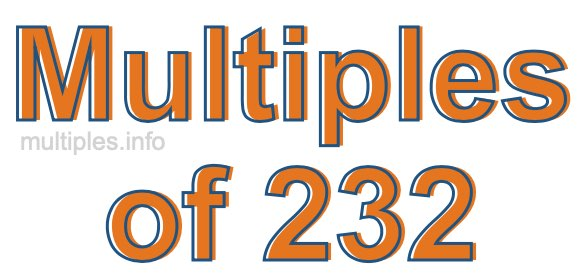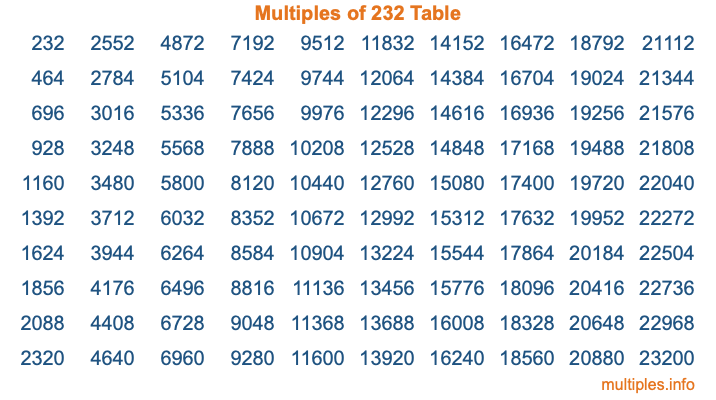Multiples of 232Welcome to the Multiples of 232 page. Here we will first teach you everything you will ever need to know about the multiples of 232, and then give you a study guide summary of everything we taught you to make sure you remember it all. Use this page to look up facts and learn information about the multiples of 232. This page will make you a multiples of two hundred thirty-two expert!

Definition of Multiples of 232
Multiples of 232 are all the numbers that when divided by 232 equal an integer. Each of the multiples of 232 are called a multiple. A multiple of 232 is created by multiplying 232 by an integer.

Therefore, to create a list of multiples of 232, you start with 1 multiplied by 232, then 2 multiplied by 232, then 3 multiplied by 232, and so on for as long as you want. Thus, the list of the first five multiples of 232 is 232, 464, 696, 928, and 1160. To see a larger list of multiples of 232, see the printable image of Multiples of 232 further down on this page. We also have a category where you can choose any nth multiple of 232.

Multiples of 232 Checker
The Multiples of 232 Checker below checks to see if any number of your choice is a multiple of 232. In other words, it checks to see if there is any number (integer) that when multiplied by 232 will equal your number. To do that, we divide your number by 232. If the the quotient is an integer, then your number is a multiple of 232.

Is  a multiple of 232?

Least Common Multiple of 232 and ...
A Least Common Multiple (LCM) is the lowest multiple that two or more numbers have in common. This is also called the smallest common multiple or lowest common multiple and is useful to know when you are adding our subtracting fractions. Enter one or more numbers below (232 is already entered) to find the LCM.

Check out our LCM Calculator if you need more details about the Least Common Multiple or if you need the LCM for different numbers for adding and subtraction fractions.

nth Multiple of 232
As we stated above, 232 is the first multiple of 232, 464 is the second multiple of 232, 696 is the third multiple of 232, and so on. Enter a number below to find the nth multiple of 232.

th multiple of 232

Multiples of 232 vs Factors of 232
232 is a multiple of 232 and a factor of 232, but that is where the similarities end. All postive multiples of 232 are 232 or greater than 232. All positive factors of 232 are 232 or less than 232.

Below is the beginning list of multiples of 232 and the factors of 232 so you can compare:

Multiples of 232: 232, 464, 696, 928, 1160, etc.

Factors of 232: 1, 2, 4, 8, 29, 58, 116, 232

As you can see, the multiples of 232 are all the numbers that you can divide by 232 to get a whole number. The factors of 232, on the other hand, are all the whole numbers that you can multiply by another whole number to get 232.

It's also interesting to note that if a number (x) is a factor of 232, then 232 will also be a multiple of that number (x).

Multiples of 232 vs Divisors of 232
The divisors of 232 are all the integers that 232 can be divided by evenly. Below is a list of the divisors of 232.

Divisors of 232: 1, 2, 4, 8, 29, 58, 116, 232

The interesting thing to note here is that if you take any multiple of 232 and divide it by a divisor of 232, you will see that the quotient is an integer.

Multiples of 232 Table
Below is an image of the first 100 multiples of 232 in a table. The table is in chronological order, column by column. The first column has the first ten multiples of 232, the second column has the next ten multiples of 232, and so on.The Multiples of 232 Table is also referred to as the 232 Times Table or Times Table of 232. You are welcome to print out our table for your studies.

Negative Multiples of 232
Although not often discussed or needed in math, it is worth mentioning that you can make a list of negative multiples of 232 by multiplying 232 by -1, then by -2, then by -3, and so on, to get the following list of negative multiples of 232:

-232, -464, -696, -928, -1160, etc.

Multiples of 232 Summary
Below is a summary of important Multiples of 232 facts that we have discussed on this page. To retain the knowledge on this page, we recommend that you read through the summary and explain to yourself or a study partner why they hold true.

There are an infinite number of multiples of 232.

A multiple of 232 divided by 232 will equal a whole number.

232 divided by a factor of 232 equals a divisor of 232.

The nth multiple of 232 is n times 232.

The largest factor of 232 is equal to the first positive multiple of 232.

232 is a multiple of every factor of 232.

232 is a multiple of 232.

A multiple of 232 divided by a divisor of 232 equals an integer.

232 divided by a divisor of 232 equals a factor of 232.

Any integer times 232 will equal a multiple of 232.

Multiples of a Number
Here you can get the multiples of another number, all with the same attention to detail as we did for multiples of 232 on this page.

Multiples of
Multiples of 233
Did you find our page about multiples of two hundred thirty-two educational? Do you want more knowledge? Check out the multiples of the next number on our list!

Copyright  |   Privacy Policy  |   Disclaimer  |   Contact